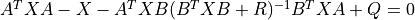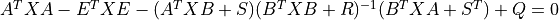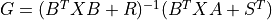# control.dare¶

control.dare(A, B, Q, R, S=None, E=None, stabilizing=True, method=None, A_s='A', B_s='B', Q_s='Q', R_s='R', S_s='S', E_s='E')[source]

Solves the discrete-time algebraic Riccati equation

X, L, G = dare(A, B, Q, R) solveswhere A and Q are square matrices of the same dimension. Further, Q is a symmetric matrix. The function returns the solution X, the gain matrix G = (B^T X B + R)^-1 B^T X A and the closed loop eigenvalues L, i.e., the eigenvalues of A - B G.

X, L, G = dare(A, B, Q, R, S, E) solves the generalized discrete-time algebraic Riccati equationwhere A, Q and E are square matrices of the same dimension. Further, Q and R are symmetric matrices. If R is None, it is set to the identity matrix. The function returns the solution X, the gain matrixand the closed loop eigenvalues L, i.e., the (generalized) eigenvalues of A - B G (with respect to E, if specified).

Parameters
• A (2D arrays) – Input matrices for the Riccati equation

• B (2D arrays) – Input matrices for the Riccati equation

• Q (2D arrays) – Input matrices for the Riccati equation

• R (2D arrays, optional) – Input matrices for generalized Riccati equation

• S (2D arrays, optional) – Input matrices for generalized Riccati equation

• E (2D arrays, optional) – Input matrices for generalized Riccati equation

• method (str, optional) – Set the method used for computing the result. Current methods are ‘slycot’ and ‘scipy’. If set to None (default), try ‘slycot’ first and then ‘scipy’.

Returns

• X (2D array (or matrix)) – Solution to the Ricatti equation

• L (1D array) – Closed loop eigenvalues

• G (2D array (or matrix)) – Gain matrix

Notes

The return type for 2D arrays depends on the default class set for state space operations. See `use_numpy_matrix()`.